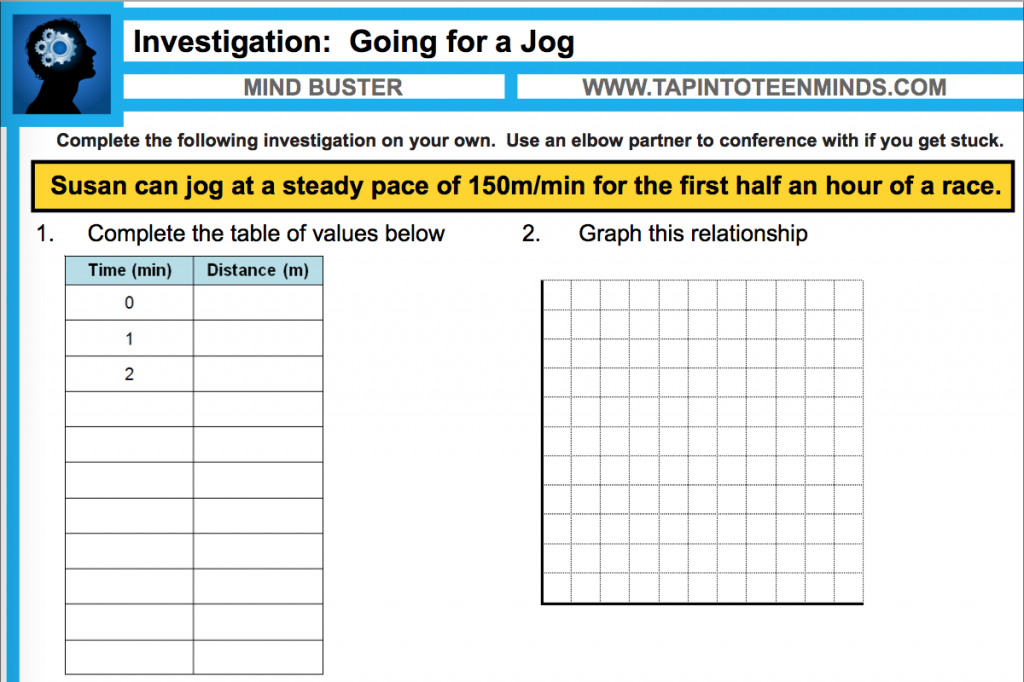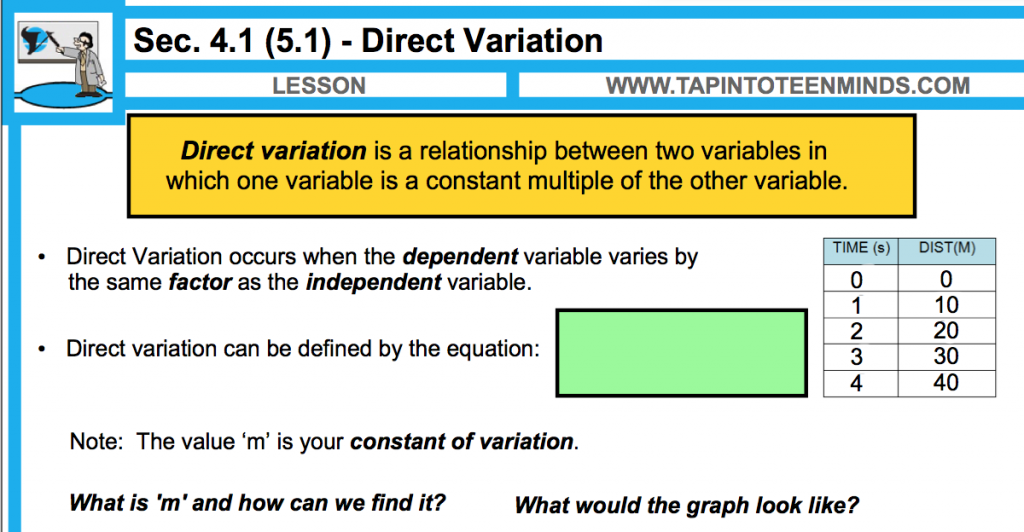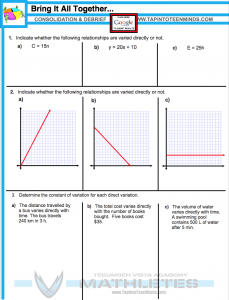# MPM1D – 4.1 – Direct Variation

#### Expectations Covered This Section:

• construct tables of values, graphs, and equations, using a variety of tools (e.g., graphing calculators, spreadsheets, graphing software, paper and pencil), to represent linear relations derived from descriptions of realistic situations;
• compare the properties of direct variation and partial variation in applications, and identify the initial value (e.g., for a relation described in words, or represented as a graph or an equation);

### Minds On

#### Investigation: Going for a JogStudents will work in their table groups to create a table of values and graph the relationship on a grid. They will then complete the following related to the problem:

Identify the independent/dependent variables.

Describe the shape of the graph.
Where does it intersect the vertical axis?

Write an equation to find the distance,  d, in metres, that Susan jogs in t mins.

Use the equation to determine the  distance that Susan can jog in 25 mins.

Consider the distance Susan jogged in 5 minutes.
What happens to this distance when the time is doubled?
What happens to the distance when the time is tripled?

The class will engage in a discussion using Apple TV as a means to quickly display different student work from across each table group.

### Action

#### Unit 4 Modelling With Graphs – 4.1 – Direct VariationThe teacher will lead a discussion about direct variation and attempt to demystify the definition:

A Direct Variation is a relationship between two variables in which one variable is a constant multiple of the other variable.

The discussion will cover the following direct variation topics:

• How we can relate direct variation to proportional reasoning,
• what a direct variation looks like in a table and graph, and
• how to find the constant of variation, m.

#### What is Direct Variation Video

A video encapsulating most of the topics covered in our classroom discussion about Direct Variation.

The teacher will scaffold the students through the lesson using a gradual release of responsibility approach. The teacher will begin the first two tasks with the students and then let groups solve the problems in the way they feel most comfortable.

The direct variation task questions target the students’ ability to identify a direct variation in a word problem, from an equation, table and graph.

Tasks can be shared out via Apple TV to show multiple methods of solving each task question.

### Consolidate

#### Identifying Direct Variation and Determining the Constant of VariationStudents will answer the consolidation questions that indicate whether students can identify a direct variation in the form of an equation, table and a graph as well as whether they can determine the constant of variation when a linear equation is a direct variation.

When complete, students will submit their consolidation answers in the Google Drive Form below:

#### MPM1D – 4.1 – Direct Variation

All Unit 4 Videos can be found on the Math Videos – Unit 4 Modelling With Graphs page.

Videos recorded before, during or after class will be listed below:

Questions From Homework:

In the following video, Mr. Pearce and the class discuss a question from the homework. This was recorded the next day at the beginning of class with the help of the students. The question asked is:

The cost of oranges varies directly with the total mass bought. 2 kg of oranges costs \$4.50.

a) Describe the relationship in words.
b) Write an equation relating the cost and the mass of the oranges. What does the constant of variation represent?
c) What is the cost of 30 kg of oranges?